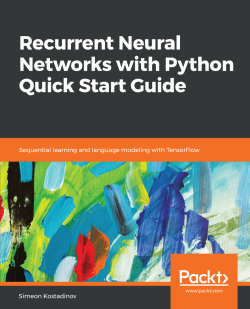### Recurrent Neural Networks with Python Quick Start Guide3.7 (3 reviews total)
• FREE Subscribe Access now
• £22.99 Print + eBook
• £15.99 eBook
• Constantly updated with 100+ new titles each month
• Breadth and depth in over 1,000+ technologies

Developers struggle to find an easy-to-follow learning resource for implementing Recurrent Neural Network (RNN) models. RNNs are the state-of-the-art model in deep learning for dealing with sequential data. From language translation to generating captions for an image, RNNs are used to continuously improve results. This book will teach you the fundamentals of RNNs, with example applications in Python and the TensorFlow library. The examples are accompanied by the right combination of theoretical knowledge and real-world implementations of concepts to build a solid foundation of neural network modeling.

Your journey starts with the simplest RNN model, where you can grasp the fundamentals. The book then builds on this by proposing more advanced and complex algorithms. We use them to explain how a typical state-of-the-art RNN model works. From generating text to building a language translator, we show how some of today's most powerful AI applications work under the hood.

After reading the book, you will be confident with the fundamentals of RNNs, and be ready to pursue further study, along with developing skills in this exciting field.

Publication date:
November 2018
Publisher
Packt
Pages
122
ISBN
9781789132335

# Introducing Recurrent Neural Networks

This chapter will introduce you to the theoretical side of the recurrent neural network (RNN) model. Gaining knowledge about what lies behind this powerful architecture will give you a head start on mastering the practical examples that are provided later in the book. Since you may often find yourself in a situation where a critical decision for your application is needed, it is essential to be aware of the building parts of this model. This will help you act appropriately for the situation.

The prerequisite knowledge for this chapter includes basic linear algebra (matrix operations). A basic knowledge in deep learning and neural networks is also a plus. If you are new to that field, I would recommend first watching the great series of videos made by Andrew Ng (https://www.youtube.com/playlist?list=PLkDaE6sCZn6Ec-XTbcX1uRg2_u4xOEky0); they will help you make your first steps so you are prepared to expand your knowledge. After reading the chapter, you will be able to answer questions such as the following:

• What is an RNN?
• Why is an RNN better than other solutions?
• How do you train an RNN?
• What are some problems with the RNN model?

# What is an RNN?

An RNN is one powerful model from the deep learning family that has shown incredible results in the last five years. It aims to make predictions on sequential data by utilizing a powerful memory-based architecture.

But how is it different from a standard neural network? A normal (also called feedforward) neural network acts like a mapping function, where a single input is associated with a single output. In this architecture, no two inputs share knowledge and the each moves in only one direction—starting from the input nodes, passing through hidden nodes, and finishing at the output nodes. Here is an illustration of the aforementioned model: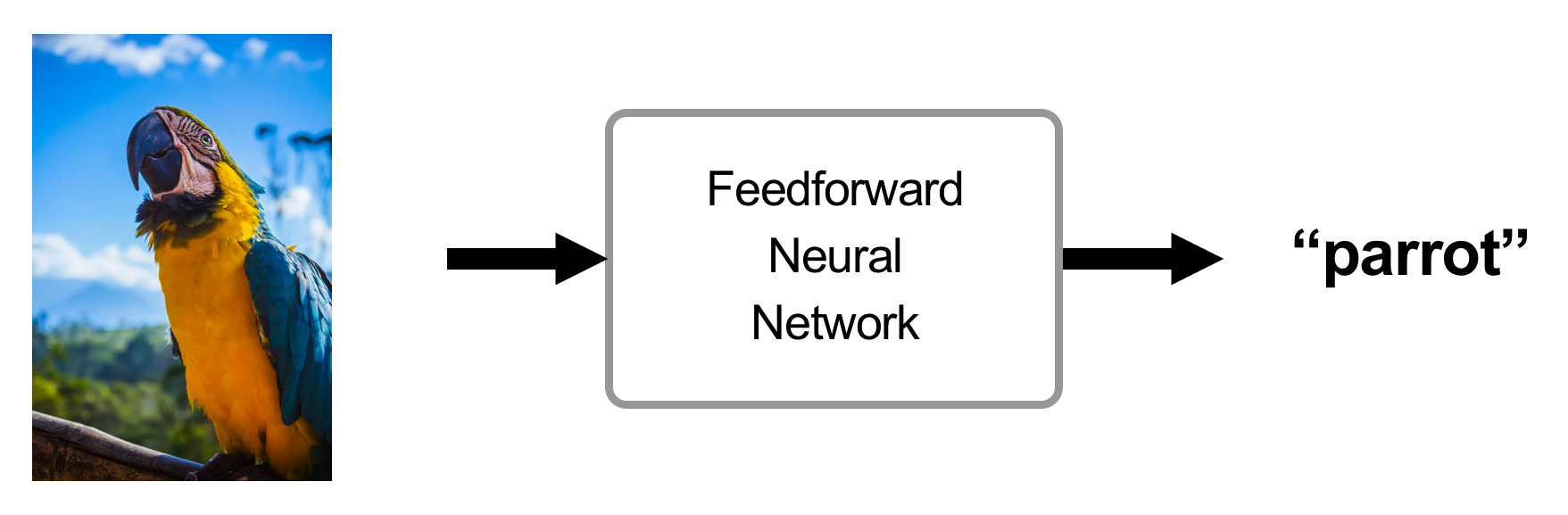On the contrary, a recurrent (also called feedback) neural network uses an additional memory state. When an input A1 (word I) is added, the network produces an output B1 (word love) and stores information about the input A1 in the memory state. When the next input A2 (word love) is added, the network produces the associated output B2 (word to) with the help of the memory state. Then, the memory state is updated using information from the new input A2. This operation is repeated for each input: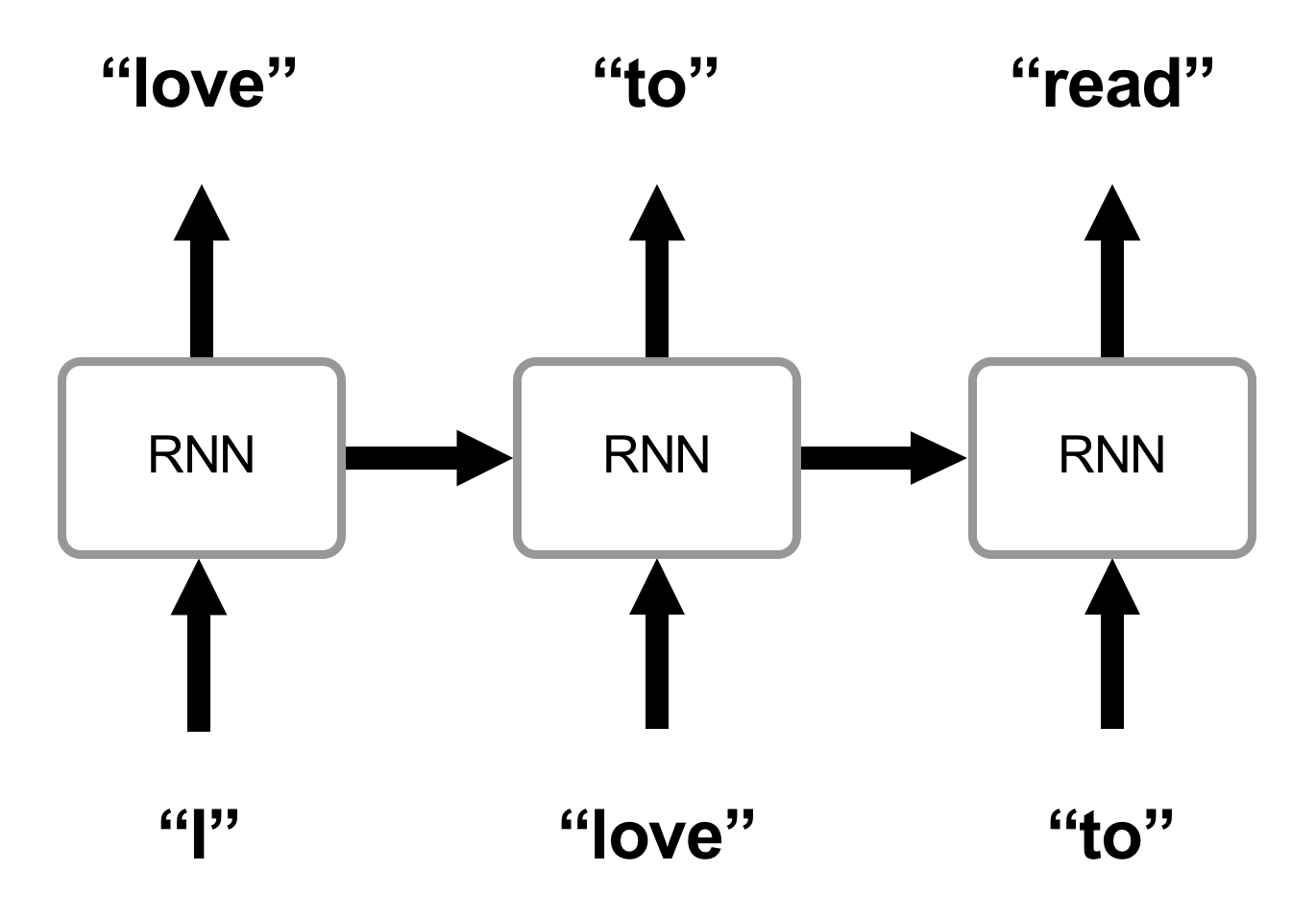You can see how with this method our predictions depend not only on the current input, but also on previous data. This is the reason why RNNs are the state-of-the-art model for dealing with sequences. Let's illustrate this with some examples.

A typical use case for the feedforward architecture is image recognition. We can see its application in agriculture for analyzing plants, in healthcare for diagnosing diseases, and in driverless cars for detecting pedestrians. Since no output in any of these examples requires specific information from a previous input, the feedforward network is a great fit for such problems.

There is also another set of problems, which are based on sequential data. In these cases, predicting the next element in the sequence depends on all the previous elements. The following is a list of several examples:

• Translating text to speech
• Predicting the next word in a sentence
• Converting audio to text
• Language translation
• Captioning videos

RNNs were first introduced in the 1980s with the invention of the Hopfield network. Later, in 1997, Hochreiter and Schmidhuber proposed an advanced RNN model called long short-term memory (LSTM). It aims to solve some major issues with the simplest recurrent neural network model, which we will reveal later in the chapter. A more recent improvement to the RNN family was presented in 2014 by Chung et al. This new architecture, called Gated Recurrent Unit, solves the same problem as LSTM but in a simpler manner.

In the next chapters of this book, we will go over the aforementioned models and see how they work and why researchers and large companies are using them on a daily basis to solve fundamental problems.

# Comparing recurrent neural networks with similar models

In recent years, RNNs, similarly to any neural network model, have become widely popular due to the easier access to large amounts of structured data and increases in computational power. But researchers have been solving sequence-based problems for decades with the help of other methods, such as the Hidden Markov Model. We will briefly compare this technique to an RNNs and outline the benefits of both approaches.

The Hidden Markov Model (HMM) is a probabilistic sequence model that aims to assign a label (class) to each element in a sequence. HMM computes the probability for each possible sequence and picks the most likely one.

Both the HMM and RNN are powerful models that yield phenomenal results but, depending on the use case and resources available, RNN can be much more effective.

# Hidden Markov model

The following are the pros and cons of a Hidden Markov Model when solving sequence-related tasks:

• Pros: Less complex to implement, works faster and as efficiently as RNNs on problems of medium difficulty.
• Cons: HMM becomes exponentially expensive with the desire to increase accuracy. For example, predicting the next word in a sentence may depend on a word from far behind. HMM needs to perform some costly operations to obtain this information. That is the reason why this model is not ideal for complex tasks that require large amounts of data.
These costly operations include calculating the probability for each possible element with respect to all the previous elements in the sequence.

# Recurrent neural network

The following are the pros and cons of a recurrent neural network when solving sequence-related tasks:

• Pros: Performs significantly better and is less expensive when working on complex tasks with large amounts of data.
• Cons: Complex to build the right architecture suitable for a specific problem. Does not yield better results if the prepared data is relatively small.

As a result of our observations, we can state that RNNs are slowly replacing HMMs in the majority of real-life applications. One ought to be aware of both models, but with the right architecture and data, RNNs often end up being the better choice.

Nevertheless, if you are interested in learning more about hidden Markov models, I strongly recommend going through some video series (https://www.youtube.com/watch?v=TPRoLreU9lA) and papers of example applications, such as Introduction to Hidden Markov Models by Degirmenci (Harvard University) (https://scholar.harvard.edu/files/adegirmenci/files/hmm_adegirmenci_2014.pdf) or Issues and Limitations of HMM in Speech Processing: A Survey (https://pdfs.semanticscholar.org/8463/dfee2b46fa813069029149e8e80cec95659f.pdf).

# Understanding how recurrent neural networks work

With the use of a memory state, the RNN architecture perfectly addresses every sequence-based problem. In this section of the chapter, we will go over a full explanation of how this works. You will obtain knowledge about the general characteristics of a neural network as well as what makes RNNs special. This section emphasizes on the theoretical side (including mathematical equations), but I can assure you that once you grasp the fundamentals, any practical example will go smoothly.

To make the explanations understandable, let's discuss the task of generating text and, in particular, producing a new chapter based on one of my favorite book series, The Hunger Games, by Suzanne Collins.

# Basic neural network overview

At the highest level, a neural network, which solves supervised problems, works as follows:

1. Obtain training data (such as images for image recognition or sentences for generating text)
2. Encode the data (neural networks work with numbers so a numeric representation of the data is required)
3. Build the architecture of your neural network model
4. Train the model until you are satisfied with the results
5. Evaluate your model by making a fresh new prediction

Let's see how these steps are applied for an RNN.

# Obtaining data

For the problem of generating a new book chapter based on the book series The Hunger Games, you can extract the text from all books in The Hunger Games series (The Hunger Games, Mockingjay, and Catching Fire) by copying and pasting it. To do that, you need to find the books, content online.

# Building the architecture

Each neural network consists of three sets of layers—input, hidden, and output. There is always one input and one output layer. If the neural network is deep, it has multiple hidden layers: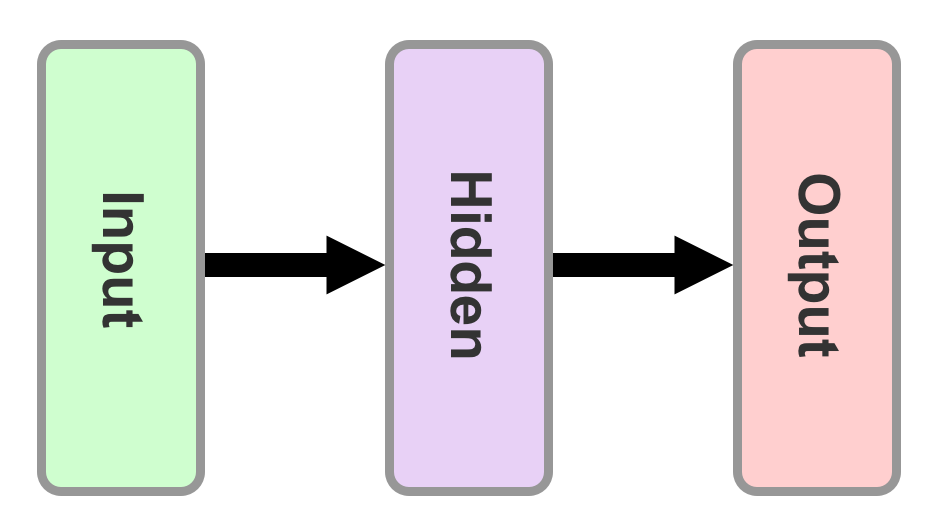The difference between an RNN and the standard feedforward network comes in the cyclical hidden states. As seen in the following diagram, recurrent neural networks use cyclical hidden states. This way, data propagates from one time step to another, making each one of these steps dependent on the previous: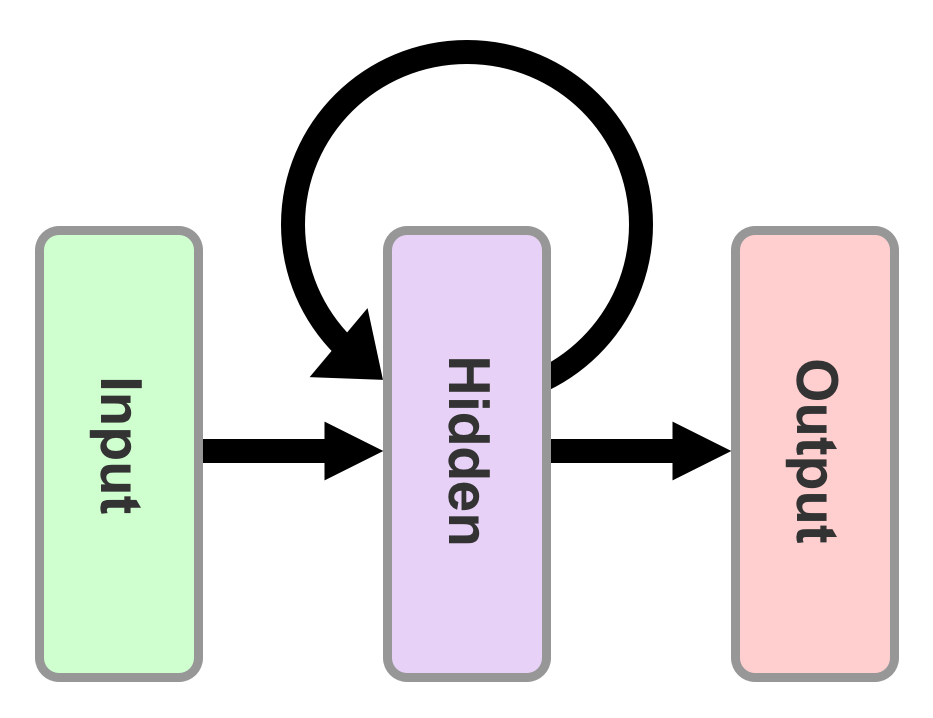A common practice is to unfold the preceding diagram for better and more fluent understanding. After rotating the illustration vertically and adding some notations and labels, based on the example we picked earlier (generating a new chapter based on The Hunger Games books), we end up with the following diagram: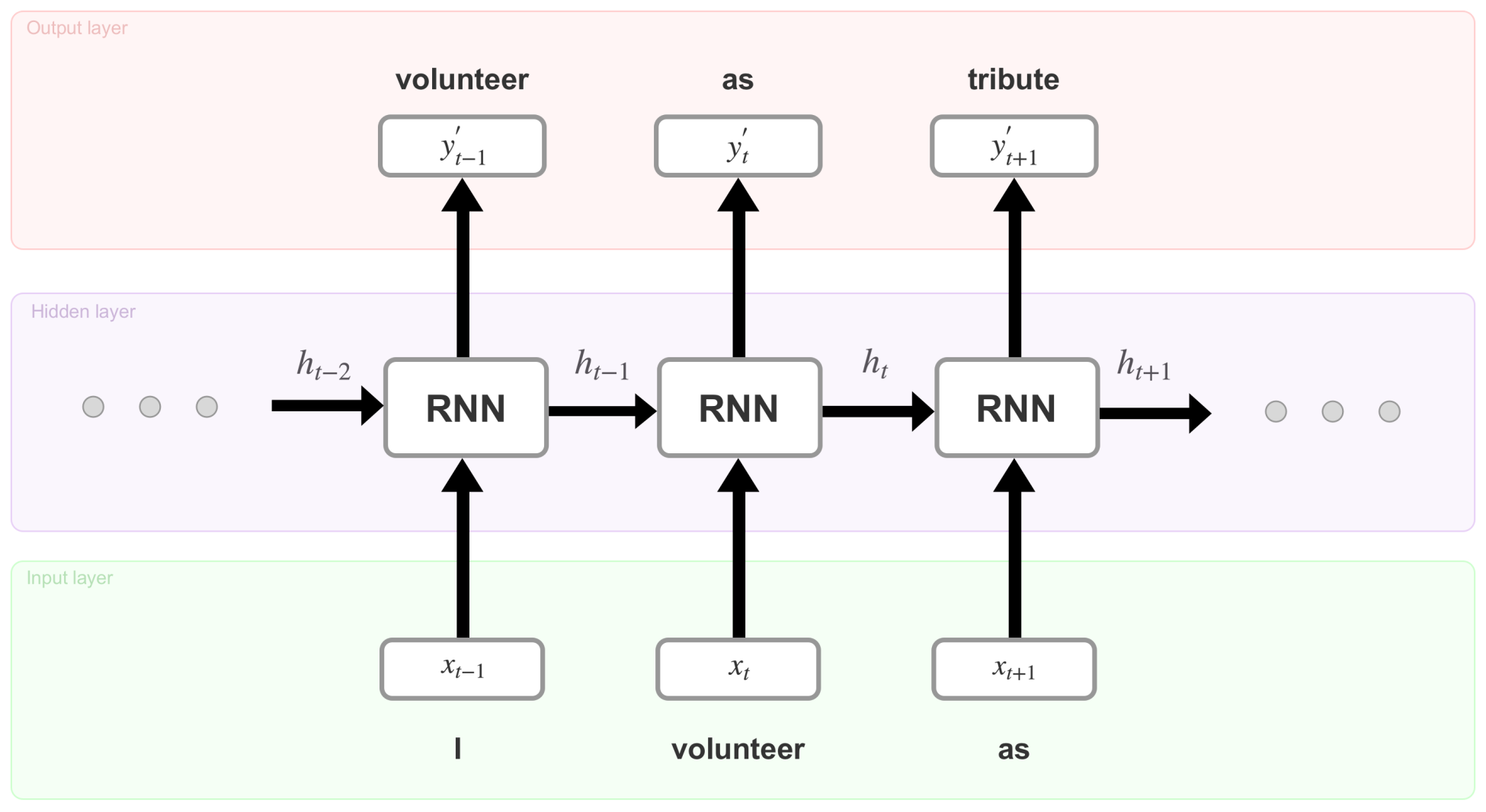This is an unfolded RNN with one hidden layer. The identically looking sets of (input + hidden RNN unit + output) are actually the different time steps (or cycles) in the RNN. For example, the combination of+ RNN +illustrates what is happening at time step. At each time step, these operations perform as follows:

1. The network encodes the word at the current time step (for example, t-1) using any of the word embedding techniques and produces a vector(The produced vector can beordepending on the specific time step)
2. Then,, the encoded version of the input word I at time step t-1, is plugged into the RNN cell (located in the hidden layer). After several equations (not displayed here but happening inside the RNN cell), the cell produces an outputand a memory state. The memory state is the result of the inputand the previous value of that memory state. For the initial time step, one can assume thatis a zero vector
3. Producing the actual word (volunteer) at time step t-1 happens after decoding the outputusing a text corpus specified at the beginning of the training
4. Finally, the network moves multiple time steps forward until reaching the final step where it predicts the word

You can see how each one of {…,, …} holds information about all the previous inputs. This makes RNNs very special and really good at predicting the next unit in a sequence. Let's now see what mathematical equations sit behind the preceding operations.

Text corpus—an array of all words in the example vocabulary.

# Training the model

All the magic in this model lies behind the RNN cells. In our simple example, each cell presents the same equations, just with a different set of variables. A detailed version of a single cell looks like this: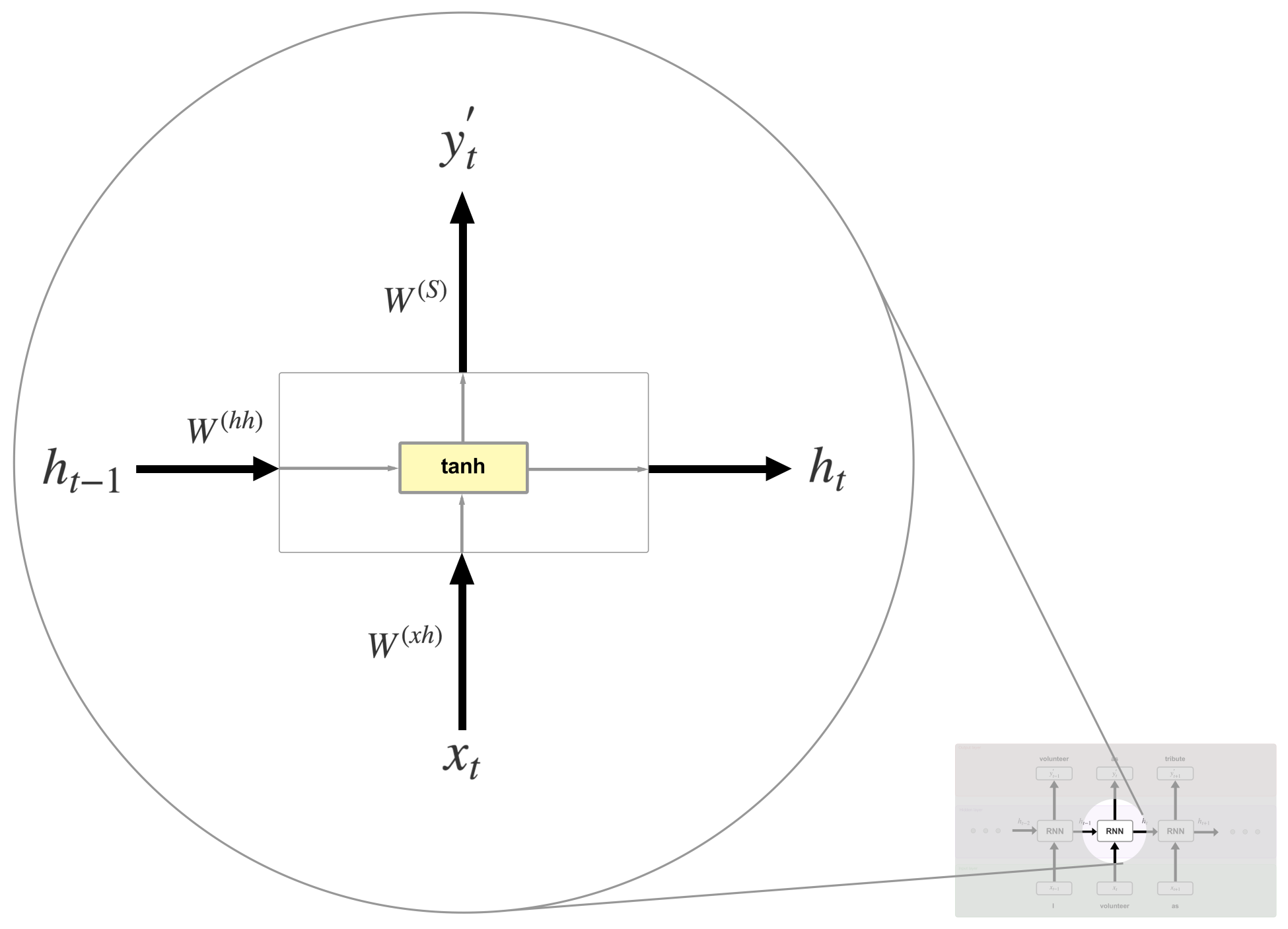First, let's explain the new terms that appear in the preceding diagram:

• Weights (,,): A weight is a matrix (or a number) that represents the strength of the value it is applied to. For example,determines how much of the inputshould be considered in the following equations.
Ifconsists of high values, thenshould have significant influence on the end result. The weight values are often initialized randomly or with a distribution (such as normal/Gaussian distribution). It is important to be noted that,  andare the same for each step. Using the backpropagation algorithm, they are being modified with the aim of  producing accurate predictions
• Biases (,): An offset vector (different for each layer), which adds a change to the value of the output• Activation function (tanh): This determines the final value of the current memory stateand the output. Basically, the activation functions map the resultant values of several equations similar to the following ones into a desired range: (-1, 1) if we are using the tanh function, (0, 1) if we are using sigmoid function, and (0, +infinity) if we are using ReLu (https://ai.stackexchange.com/questions/5493/what-is-the-purpose-of-an-activation-function-in-neural-networks)

Now, let's go over the process of computing the variables. To calculateand, we can do the following:As you can see, the memory stateis a result of the previous valueand the input. Using this formula helps in retaining information about all the previous states.

The inputis a one-hot representation of the word volunteer. Recall from before that one-hot encoding is a type of word embedding. If the text corpus consists of 20,000 unique words and volunteer is the 19th word, thenis a 20,000-dimensional vector where all elements are 0 except the one at the 19th position, which has a value of 1, which suggests that we only taking into account this particular word.

The sum between, andis passed to the tanh activation function, which squashes the result between -1 and 1 using the following formula:In this, e = 2.71828 (Euler's number) and z is any real number.

The outputat time step t is calculated usingand the softmax function. This function can be categorized as an activation with the exception that its primary usage is at the output layer when a probability distribution is needed. For example, predicting the correct outcome in a classification problem can be achieved by picking the highest probable value from a vector where all the elements sum up to 1. Softmax produces this vector, as follows:In this, e = 2.71828 (Euler's number) and z is a K-dimensional vector. The formula calculates probability for the value at the ith position in the vector z.

After applying the softmax function,becomes a vector of the same dimension as(the corpus size 20,000) with all its elements having a total sum of 1. With that in mind, finding the predicted word from the text corpus becomes straightforward.

# Evaluating the model

Once an assumption for the next word in the sequence is made, we need to assess how good this prediction is. To do that, we need to compare the predicted wordwith the actual word from the training data (let's call it). This operation can be accomplished using a loss (cost) function. These types of functions aim to find the error between predicted and actual values. Our choice will be the cross-entropy loss function, which looks like this:Since we are not going to give a detailed explanation of this formula, you can treat it as a black box. If you are curious about how it works, I recommend reading the article Improving the way neural networks work by Michael Nielson (http://neuralnetworksanddeeplearning.com/chap3.html#introducing_the_cross-entropy_cost_function). A useful thing to know is that the cross-entropy function performs really well on classification problems.

After computing the error, we came to one of the most complex and, at the same time, powerful techniques in deep learning, called backpropagation.

In simple terms, we can state that the backpropagation algorithm traverses backward through all (or several) time steps while updating the weights and biases of the network. After repeating this procedure, and a certain amount of training steps, the network learns the correct parameters and will be able to yield better predictions.

To clear out any confusion, training and time steps are completely different terms. In one time step, we get a single element from the sequence and predict the next one. A training step is composed of multiple time steps where the number of time steps depends on how large the sequence for this training step is. In addition, time steps are only used in RNNs, but training ones are a general neural network concept.

After each training step, we can see that the value from the loss function decreases. Once it crosses a certain threshold, we can state that the network has successfully learned to predict new words in the text.

The last step is to generate the new chapter. This can happen by choosing a random word as a start (such as: games) and then predicting the next words using the preceding formulas with the pre-trained weights and biases. Finally, we should end up with somewhat meaningful text.

# Key problems with the standard recurrent neural network model

Hopefully, now you have a good understanding of how a recurrent neural network works. Unfortunately, this simple model fails to make good predictions on longer and complex sequences. The reason behind this lies in the so-called vanishing/exploding gradient problem that prevents the network from learning efficiently.

As you already know, the training process updates the weights and biases using the backpropagation algorithm. Let's dive one step further into the mathematical explanations. In order to know how much to adjust the parameters (weights and biases), the network computes the derivative of the loss function (at each time step) with respect to the current value of these parameters. When this operation is done for multiple time steps with the same set of parameters, the value of the derivative can become too large or too small. Since we use it to update the parameters, a large value can result in undefined weights and biases and a small value can result in no significant update, and thus no learning.

Derivative is a way to show the rate of change; that is, the amount by which a function is changing at one given point. In our case, this is the rate of change of the loss function with respect to the given weights and biases.

This issue was first addressed by Bengio et al. in 1994, which led to an introduction of the LSTM network with the aim of solving the vanishing/exploding gradient problem. Later in the book, we will reveal how LSTM does this in an excellent fashion. Another model, which also overcomes this challenge, is the gated recurrent unit. In Chapter 3, Generating Your Own Book Chapter, you will see how this is being done.

For more information on the vanishing/exploding gradient problem, it would be useful to go over Lecture 8 from the course Natural Language Processing with Deep Learning by Stanford University (https://www.youtube.com/watch?v=Keqep_PKrY8) and the paper On the difficulty of training recurrent neural networks (http://proceedings.mlr.press/v28/pascanu13.pdf).

# Summary

In this chapter, we introduce the recurrent neural network model using theoretical explanations together with a particular example. The aim is to grasp the fundamentals of this powerful system so you can understand the programming exercises better. Overall, the chapter included the following:

• A brief introduction to RNNs
• The difference between RNNs and other popular models
• Illustrating the use of RNNs through an example
• The main problems with a standard RNN

In the next chapter, we will go over our first practical exercise using recurrent neural networks. You will get to know the popular TensorFlow library, which makes it easy to build machine learning models. The next section will give you a nice first hands-on experience and prepare you for solving more difficult problems.